# Mutual Induction

Whenever the primary circuit is switched on or off deflection is observed in the secondary circuit. At the time of switching on or the circuit the current in primary various which produces varying flux which is linked with secondary to EMF is induced in it. The phenomenon due to which can EMF is induced in a coil when a varying current flows in adjoining coil is known as mutual induction.

If Ip is the current flowing I primary and ɸ as the flux linked with secondary then we have

ɸs  x  Ip

ɸs  = m. Ip

m is constant of proportionality known as coefficient of mutual induction.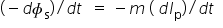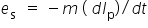Unit of mutual inductance :- ‘henry’

M = 1 henry if ls es is equal to 1V when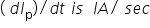Expression for mutual inductance of two coils,

ɸs  = Ns BA

ɸs  = n2 l  BA

ɸs  = n2 l μo BA n1 Ip A

ɸs  = μo n1 n2 l A Ip

but        ɸs  =m.Ip

m= μo n1 n2 l A

m depends upon physical parameters of two coils it is independent of the flux and current above relation is valid  when (1) flue linkage is 100% m depends upon the (2) relative position of ht two coils (3) m would be large if flux linked is large.

Relation between self inductance and mutual inductance.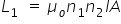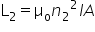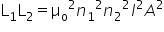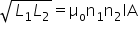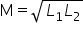The above relation gives maximum value of mutual inductance when flux linked is 100% in general mutual inductance b/w 2 coils is given by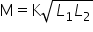K = is a constant known as coupling constant

Value of K depends upon the relative position of the coils.

For 100% flux linkage    K = 1

For independent coils   K = 0

For other situation      K lies b/w   0 and 1

Related Keywords